• ## 向量点乘与叉乘

万次阅读 多人点赞 2019-02-25 14:06:01
向量点乘与叉乘 向量（Vector）  在几乎所有的几何问题中，向量（有时也称矢量）是一个基本点。向量的定义包含方向和一个数（长度）。在二维空间中，一个向量可以用一对x和y来表示。例如由点（1,3）到（5,1的向量...
向量点乘与叉乘
向量（Vector）       在几乎所有的几何问题中，向量（有时也称矢量）是一个基本点。向量的定义包含方向和一个数（长度）。在二维空间中，一个向量可以用一对x和y来表示。例如由点（1,3）到（5,1的向量可以用(4,-2）来表示。这里大家要特别注意，我这样说并不代表向量定义了起点和终点。向量仅仅定义方向和长度。 向量加法       向量也支持各种数学运算。最简单的就是加法。我们可以对两个向量相加，得到的仍然是一个向量。我们有：       V1（x1, y1）+V2（x2, y2）=V3(x1+x2, y1+y2)       下图表示了四个向量相加。注意就像普通的加法一样,相加的次序对结果没有影响（满足交换律），减法也是一样的。  点乘（Dot Product）       如果说加法是凭直觉就可以知道的，另外还有一些运算就不是那么明显的，比如点乘和叉乘。       点乘比较简单，是相应元素的乘积的和：       V1( x1, y1)   V2(x2, y2) = x1*x2 + y1*y2       注意结果不是一个向量，而是一个标量（Scalar）。点乘有什么用呢，我们有：       A   B = |A||B|Cos(θ)       θ是向量A和向量B见的夹角。这里|A|我们称为向量A的模(norm)，也就是A的长度， 在二维空间中就是|A| = sqrt(x2+y2)。这样我们就和容易计算两条线的夹角：    Cos(θ) = AB /(|A||B|)       当然你知道要用一下反余弦函数acos()啦。（回忆一下cos(90)=0 和cos(0) = 1还是有好处的，希望你没有忘记。）这可以告诉我们如果点乘的结果，简称点积，为0的话就表示这两个向量垂直。当两向量平行时，点积有最大值       另外，点乘运算不仅限于2维空间，他可以推广到任意维空间。（译注：不少人对量子力学中的高维空间无法理解，其实如果你不要试图在视觉上想象高维空间，而仅仅把它看成三维空间在数学上的推广，那么就好理解了）叉乘（cross product）       相对于点乘，叉乘可能更有用吧。2维空间中的叉乘是：       V1(x1, y1) X V2(x2, y2) = x1y2 – y1x2       看起来像个标量，事实上叉乘的结果是个向量，方向在z轴上。上述结果是它的模。在二维空间里，让我们暂时忽略它的方向，将结果看成一个向量，那么这个结果类似于上述的点积，我们有：     A x B = |A||B|Sin(θ)
然而角度 θ和上面点乘的角度有一点点不同，他是有正负的，是指从A到B的角度。因此 ，向量的外积不遵守乘法交换率，因为向量a×向量b=-向量b×向量a在物理学中，已知力与力臂求外积，就是向量的外积，即叉乘。
向量c的方向与a,b所在的平面垂直，且方向要用“右手法则”判断。判断方法如下：
1.右手手掌张开，四指并拢，大拇指垂直于四指指向的方向；
2.伸出右手，四指弯曲，四指与A旋转到B方向一致，那么大拇指指向为C向量的方向。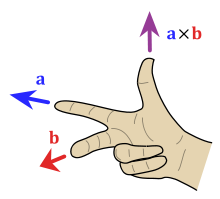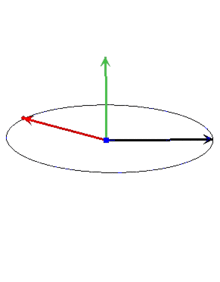另外还有一个有用的特征那就是叉积的绝对值就是A和B为两边说形成的平行四边形的面积。也就是AB所包围三角形面积的两倍。在计算面积时，我们要经常用到叉积。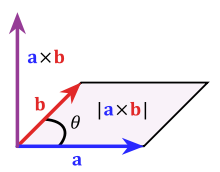（译注：三维及以上的叉乘参看维基：http://en.wikipedia.org/wiki/Cross_product）点-线距离       找出一个点和一条线间的距离是经常遇见的几何问题之一。假设给出三个点，A，B和C，你想找出点C到点A、B定出的直线间距离。第一步是找出A到B的向量AB和A到C的向量AC，现在我们用该两向量的叉积除以|AB|，这就是我们要找的的距离了（下图中的红线）。     d = (AB x AC)/|AB|        如果你有基础的高中几何知识，你就知道原因了。上一节我们知道(AB X AC)/2是三角形ABC的面积，这个三角形的底是|AB|，高就是C到AB的距离。有时叉积得到的是一个负值，这种情况下距离就是上述结果的绝对值。       当我们要找点到线段的距离时，情况变得稍稍复杂一些。这时线段与点的最短距离可能是点到线段的某一端点，而不是点到直线的垂线。例如上图中点C到线段AB的最短距离应该是线段BC。我们有集中不同的方法来判断这种特殊情况。第一种情况是计算点积AB B来判定两线段间夹角。如果点积大于等于零，那么表示AB到BC是在-90到90度间，也就是说C到AB的垂线在AB外，那么AB上到C距离最近的点就是B。同样，如果BAAC大于等于零，那么点A就是距离C最近的点。如果两者均小于零，那么距离最近的点就在线段AB中的莫一点。 源代码参考如下：      //Compute the dot product AB   BC      int dot(int[] A, int[] B, int[] C){          AB = new int;          BC = new int;          AB = B-A;          AB = B-A;          BC = C-B;          BC = C-B;          int dot = AB * BC + AB * BC;          return dot;      }      //Compute the cross product AB x AC      int cross(int[] A, int[] B, int[] C){          AB = new int;          AC = new int;          AB = B-A;          AB = B-A;          AC = C-A;          AC = C-A;          int cross = AB * AC - AB * AC;          return cross;      }      //Compute the distance from A to B      double distance(int[] A, int[] B){          int d1 = A - B;          int d2 = A - B;          return sqrt(d1*d1+d2*d2);      }      //Compute the distance from AB to C      //if isSegment is true, AB is a segment, not a line.      double linePointDist(int[] A, int[] B, int[] C, boolean isSegment){          double dist = cross(A,B,C) / distance(A,B);          if(isSegment){              int dot1 = dot(A,B,C);              if(dot1 > 0)return distance(B,C);              int dot2 = dot(B,A,C);              if(dot2 > 0)return distance(A,C);          }          return abs(dist);      }       上面的代码看起来似乎是很繁琐。不过我们可以看看在C++和C#中，采用了运算符重载的类point，用‘*’代表点乘，用'^'代表叉乘（当然'+''-'还是你所希望的），那么看起来就简单些，代码如下：    //Compute the distance from AB to C      //if isSegment is true, AB is a segment, not a line.      double linePointDist(point A, point B, point C, bool isSegment){          double dist = ((B-A)^(C-A)) / sqrt((B-A)*(B-A));          if(isSegment){              int dot1 = (C-B)*(B-A);              if(dot1 > 0)return sqrt((B-C)*(B-C));              int dot2 = (C-A)*(A-B);              if(dot2 > 0)return sqrt((A-C)*(A-C));          }          return abs(dist);      }
展开全文• 【参考原文】Unity3D之Vector3.Dot和Vector3.Cross的使用 【参考原文】Unity3D之点乘和差乘 　在Unity3D中，Vector3.Dot表示求两个向量的点积;...　简单的说，点乘判断角度，叉乘判断方向。　形象的说当一个敌
【参考原文】Unity3D之Vector3.Dot和Vector3.Cross的使用  【参考原文】Unity3D之点乘和差乘  　　在Unity3D中，Vector3.Dot表示求两个向量的点积;Vector3.Cross表示求两个向量的叉积。  　　  　　点积计算的结果为数值，而叉积计算的结果为向量。两者要注意区别开来。  　　  　　简单的说，点乘判断角度，叉乘判断方向。
形象的说当一个敌人在你身后的时候，叉乘可以判断你是往左转还是往右转更好的转向敌人，点乘得到你当前的面朝向的方向和你到敌人的方向的所成的角度大小。
1.点积

a·b=|a|·|b|cos＜a,b＞

|a|和|b|表示向量的模＜a,b＞表示两个向量的夹角。＜a,b＞和＜b,a＞ 夹角是不分顺序的。通过点积可以计算两个向量的夹角的。
如果两个向量a,b均 为单位 向量 ,那么a.b等于向量b在向量a方向上的投影的长度
应用
通过点积的计算我们可以简单粗略的判断当前物体是否朝向另外一个物体: 只需要计算当前物体的transform.forward向量与 (otherObj.transform.position – transform.position)的点积即可。  - 若结果 == 0，则两向量互垂直 。  - 若结果 > 0 ，则两向量夹角小于90°，当前物体面对  - 若结果 < 0 ，则两向量夹角大于 90°，当前物体背对
2.叉积

c =a x b

其中a,b,c均为向量。即两个向量的叉积得到的还是向量！性质1：c⊥a，c⊥b，即向量c垂直与向量a,b所在的平面。性质2：模长|c|=|a||b|sin＜a,b＞性质3：满足右手法则。从这点我们有axb ≠ bxa，而axb = – bxa。所以我们可以使用叉积的正负值来判断向量a，b的相对位置，即向量b是处于向量a的顺时针方向还是逆时针方向。
求两向量夹角
using UnityEngine;
using System.Collections;

public class MainScript : MonoBehaviour
{
//向量a
private Vector3 a;
//向量b
private Vector3 b;

void Start ()
{
//向量的初始化
a = new Vector3 (1, 2, 1);
b = new Vector3 (5, 6, 0);
}

void OnGUI ()
{
//点积的返回值
float c = Vector3.Dot (a, b);
//向量a,b的夹角,得到的值为弧度，我们将其转换为角度，便于查看！
float angle = Mathf.Acos (Vector3.Dot (a.normalized, b.normalized)) * Mathf.Rad2Deg;
GUILayout.Label ("向量a，b的点积为：" + c);
GUILayout.Label ("向量a，b的夹角为：" + angle);

//叉积的返回值
Vector3 e = Vector3.Cross (a, b);
Vector3 d = Vector3.Cross (b, a);
//向量a,b的夹角,得到的值为弧度，我们将其转换为角度，便于查看！
angle = Mathf.Asin (Vector3.Distance (Vector3.zero, Vector3.Cross (a.normalized, b.normalized))) * Mathf.Rad2Deg;
GUILayout.Label ("向量axb为：" + e);
GUILayout.Label ("向量bxa为：" + d);
GUILayout.Label ("向量a，b的夹角为：" + angle);
}
}  
上面的示例中，我们定义了两个向量a和b。分别求出了他们的点积和叉积，并通过点积和叉积来反过来计算他们的夹角。  这里要说明的是:  1.a.normalized 和 b.normalized 表示的是两个向量的单位向量, 因为在公式里，有向量和模的除法，得出来的结果就是单位向量（单位向量模为1），所以我们这里和后面都直接用单位向量来计算，省去不少麻烦。
3.通过叉积计算度数是通过公式|c|=|a||b|sin＜a,b＞来逆向求值。|c| 其实就是叉积的模，换句话说，也代表着Vector3.Distance (Vector3.zero, Vector3.Cross (a.normalized, b.normalized))的值。  结果图如下: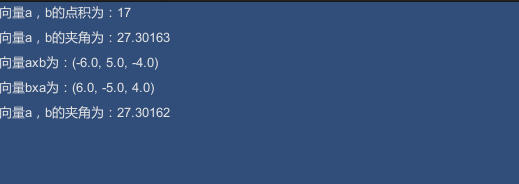点乘与叉乘项目中的应用
项目需求：  1 通过点乘计算物体B在物体A的前方还是后方  2 通过叉乘，计算物体A转向物体B时，最小角的旋转方向
实现过程：  首先计算出物体A的前方朝向向量v_Bz=B.transform.forward，然后计算物体B相对于物体A的位置向量 v_AB = A.position - B.position;
通过计算v_Bz与v_AB向量点乘结果的正负，判断物体B在物体A的前后  如果物体B在物体A前方， Vector3.Dot(v_Bz,v_AB)大于0  如果物体B在物体A后方， Vector3.Dot(v_Bz,v_AB)小于0
通过计算v_Bz与v_AB向量叉乘的结果，判断旋转方向  如果逆时针旋转，v_C = Vector3.Cross(v_Bz, v_AB)为沿y轴负方向  如果顺时针旋转，v_C = Vector3.Cross(v_Bz, v_AB)为沿y轴正方向
using UnityEngine;
using System.Collections;

public class Vector3_Dot : MonoBehaviour {

public Transform A, B;
Vector3 v_Bz, v_AB, v_A,v_B,v_C;
string str = "";

// Use this for initialization
void Start()
{

}

// Update is called once per frame
void Update()
{

//点乘
v_Bz = B.transform.forward;//B.transform.TransformDirection(Vector3.forward);
v_AB = A.position - B.position;
float f = Vector3.Dot(v_Bz,v_AB);
if (f>0)
{
str = "A在B自身座标系的前方";
}
else if (f<0)
{
str = "A在B自身座标系的后方";

}
else
{
str = "A在B自身座标系的左前方或右方";
}

//差乘
v_A = A.position;
v_B = B.position;
v_C = Vector3.Cross(v_Bz, v_AB);
// A.Rotate(0,0,0);

}

void OnGUI()
{
GUI.Label(new Rect(10,10,200,60),str);

}

void OnDrawGizmos()
{
//差乘绘制相关线
Gizmos.color = Color.blue;
Gizmos.DrawLine(-v_C,Vector3.zero);

//点乘绘制相关线
Gizmos.color = Color.yellow;
Gizmos.DrawLine(Vector3.zero,A.position);

Gizmos.color = Color.yellow;
Gizmos.DrawLine(Vector3.zero,B.position);

Gizmos.color = Color.red;
Gizmos.DrawLine(A.position, B.position);

Gizmos.color = Color.red;
Gizmos.DrawLine(Vector3.zero,v_AB);

Gizmos.color = Color.green;
// Gizmos.DrawLine(A.transform.position, Vector3.left);

}
}总结
1.判断目标在自己的前后方位可以使用下面的方法:
Vector3.Dot(transform.forward, target.position-transform.position)
返回值为正时,目标在自己的前方,反之在自己的后方
2.判断目标在机子的左右方位可以使用下面的方法:
Vector3.Cross(transform.forward, target.position-transform.position).y
返回值为正时,目标在自己的右方,反之在自己的左方
展开全文• title: 向量点乘叉乘 categories: Math tags: Math Knowledge 向量点乘叉乘 假设存在向量a和向量b:$a=[a_{1},a_{2},a_{3}],b=[b_{1},b_{2},b_{3}]$ 点乘 向量a和向量b的点乘公式如下: $a\bullet b=a_{1}...  title: 向量点乘和叉乘 categories: Math tags: MathKnowledge 向量点乘和叉乘 假设存在向量a和向量b:$ a=[a_{1},a_{2},a_{3}],b=[b_{1},b_{2},b_{3}] $ 点乘 向量a和向量b的点乘公式如下: $ a\bullet b=a_{1}b_{1}+a_{2}b_{2}+a_{3}b_{3} $ 要求是向量a和向量的b的维度要相同。 点乘的几何意义 点乘的几何意义是可以用来表征或者计算两个向量之间的夹角，以及在b向量或在a向量方向上的投影，公式如下: $ a\bullet b=a_{1}b_{1}+a_{2}b_{2}+a_{3}b_{3}=|a||b|cos \theta $ 首先假设一下向量构成: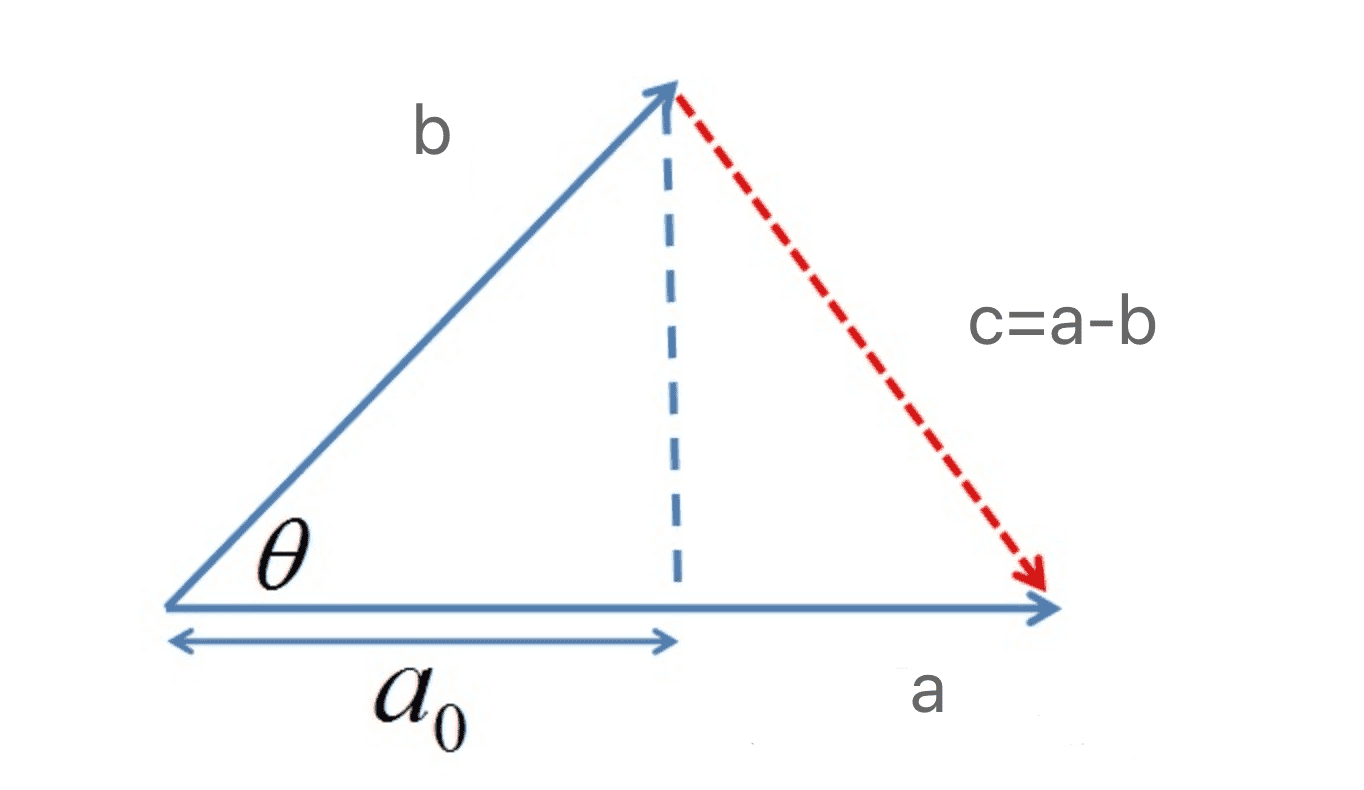推导过程如下: 根据上图可以得到c=a-b根据三角余弦定理可以得到: $ c^{2}=a^{2}+b^{2}-2|a||b|cos \theta$根据c=a-b，我们可以对上式变换得到 $ (a-b) \bullet(a-b)=a^{2}+b^{2}-2 a \bullet b=a^{2}+b^{2}-2|a \| b| \cos \theta $化简上式我们可以得到: $ a\bullet b=|a||b|cos \theta $因此在已知向量a和向量b长度的情况下，我们可以计算得到a和b的夹角θ$ \theta=arc\: cos\left ( \frac{a \bullet b}{|a||b|} \right ) $ 根据上述公式就可以计算向量a和向量b之间的夹角，从而就进一步判断出这两个向量是否是同一方向，是否正交，具体对应关系如下: a·b>0 : 方向基本相同，夹角在0到90度之间a·b=0 : 两个向量正交，相互垂直a·b<0 : 方向基本相反，夹角在90度到180度之间 叉乘 两个向量的叉乘，又叫向量积、叉积，叉乘的运算结果是一个向量而不是一个标量。并且两个向量的叉乘与这两个向量组成的坐标平面垂直 同样是使用之前假设的向量a和向量b，我们可以得到向量a和向量b的叉乘公式: $ a \times b=\left|\begin{array}{lll}\mathrm{i} & \mathrm{j} & \mathrm{k} \\a_{1} & a_{2} & a_{3} \\b_{1} & b_{2} & b_{3}\end{array}\right|=\left(a_{2}b_{3}-a_{3}b_{2}\right) i-\left(a_{1}b_{3}-a_{3}b_{1}\right) j+\left(a_{1}b_{2}-a_{2}b_{1}\right) k $ 其中$ i=(1,0,0),j=(0,1,0),k=(0,0,1) $, 其中ijk均为单位向量，最后叉乘的结果为一个向量，所以ijk要为向量，因此单纯的ab相乘再相减得到的标量。 因此根据上述i、j、k间的关系，有如下式子(直接用向量表示): $ a\times b=\left( a_{2}b_{3}-a_{3}b_{2}, a_{1}b_{3}-a_{3}b_{1}, a_{1}b_{2}-a_{2}b_{1} \right) \$
注意: 一个向量叉乘自己得到的是0向量，根据右手定则，叉乘得到的结果无论怎么旋转都会永远会垂直这个向量，因此只有0向量满足。
叉乘的几何意义
在三维几何中，向量a和向量b的叉乘结果是一个向量，这个向量又称为法向量，它是a和b构成的平面的法向量(垂直)。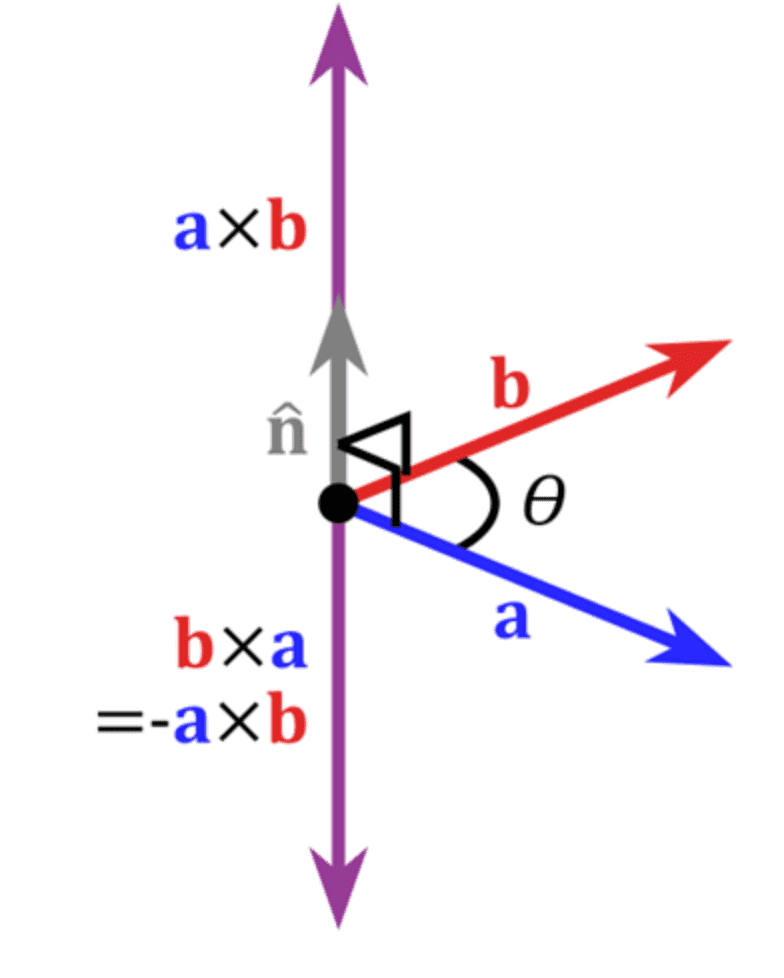如上图。
右手法则：使用右手法则来判断a×b向量的方向,如下图所示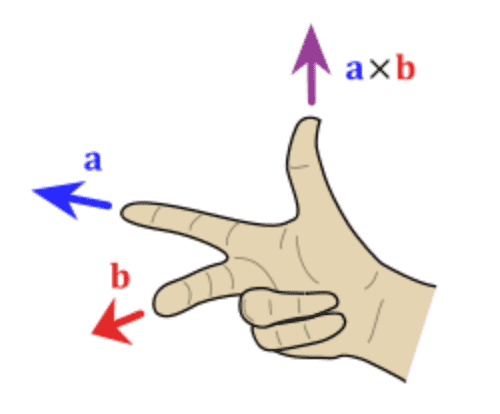判断方法如下:
右手手掌张开，四指并拢，大拇指垂直于四指指向的方向伸出右手，四指弯曲，四指与A旋转到B方向一致，那么大拇指指向为C向量的方向
展开全文线性代数 Math
• 简要介绍了向量点乘叉乘
1 点乘
向量的点乘，也叫点积、内积、数量积。是指接受在实数

R

R

上的两个向量并返回一个实数值标量的二元运算。
点积有两种定义方式：代数方式和几何方式。
1.1 代数定义
已知两个向量

a

⃗

=

[

a

1

,

a

2

,

.

.

.

,

a

n

]

\vec a=[a_1,a_2,...,a_n]

，

b

⃗

=

[

b

1

,

b

2

,

.

.

.

,

b

n

]

\vec b=[b_1,b_2,...,b_n]

，则向量

a

⃗

\vec a

与向量

b

⃗

\vec b

的内积代数定义为

a

⋅

b

=

a

1

b

1

+

a

2

b

2

+

.

.

.

+

a

n

b

n

a·b=a_1b_1+a_2b_2+...+a_nb_n

即，对应元素相乘再相加。
1.2 几何定义(只针对二维和三维空间)
已知两个向量

a

⃗

=

[

x

1

,

y

1

,

z

1

]

\vec a=[x_1,y_1,z_1]

，

b

⃗

=

[

x

2

,

y

2

,

z

2

]

\vec b=[x_2,y_2,z_2]

，|a|、|b| 分别为向量

a

⃗

\vec a

与向量

b

⃗

\vec b

的模，

<

a

,

b

>

=

θ

∈

[

0

,

π

]

<a,b>=θ∈[0,\pi]

为两向量夹角，则向量

a

⃗

\vec a

与向量

b

⃗

\vec b

的内积几何定义为：

a

⋅

b

=

∣

a

∣

∣

b

∣

c

o

s

θ

a·b=|a||b|cosθ

几何意义： 可以用来表示一个向量在另一个向量上的投影长度，为一个标量。
2 叉乘
向量的叉乘，也叫叉积、外积、向量积，是一种在向量空间中(也就是说向量元素个数为3)向量的二元运算。与点积不同，叉乘的运算结果是一个向量而不是一个标量，并且两个向量的叉积与这两个向量所构成的平面垂直。
2.1 定义
已知两个向量

a

⃗

=

[

x

1

,

y

1

,

z

1

]

\vec a=[x_1,y_1,z_1]

，

b

⃗

=

[

x

2

,

y

2

,

z

2

]

\vec b=[x_2,y_2,z_2]

，|a|、|b| 分别为向量

a

⃗

\vec a

与向量

b

⃗

\vec b

的模，

<

a

,

b

>

=

θ

∈

[

0

,

π

]

<a,b>=θ∈[0,\pi]

为两向量夹角，则向量

a

⃗

\vec a

与向量

b

⃗

\vec b

的叉积定义为：
模长：

∣

a

×

b

∣

=

∣

a

∣

∣

b

∣

s

i

n

θ

|a×b|=|a||b|sinθ

方向：两个向量的叉积与这两个向量所构成的平面垂直，且遵循右手准则（右手的四指从a以不超过180°的转角转向b时，竖起的大拇指指向是叉乘的方向。）
2.2 坐标运算
已知两个向量

a

⃗

=

[

x

1

,

y

1

,

z

1

]

\vec a=[x_1,y_1,z_1]

，

b

⃗

=

[

x

2

,

y

2

,

z

2

]

\vec b=[x_2,y_2,z_2]

，则

a

⃗

×

b

⃗

=

∣

i

j

k

x

1

y

1

z

1

x

2

y

2

z

2

∣

\vec a×\vec b=\begin{vmatrix} i&j&k\\ x_1&y_1&z_1\\ x_2&y_2&z_2\\ \end{vmatrix}

i

、

j

、

k

i、j、k

为XYZ三个轴的单位向量
展开全文线性代数
• 你讲获得向量点乘叉乘、矩阵乘法和np.dot的技能
• 首先明显的区别在于：两个向量点乘的结果是一个标量，而两个向量叉乘的结果则还是一个向量。如下面的例子： 点乘： 向量a = (a1, a2, a3), 是一个1行3列的向量。向量b=(b1, 是一个3行1列的向量。两者点乘的结果为 ...
• 结果为一个值，可以看出两个向量点乘结果的正负值取决于它们的夹角，这个非常有用；比如通过这个夹角可以判断摄像机向量和物体顶点法线向量的点乘结果，如果为正说明顶点在正面，如果为负说明顶点在反面（可以剔除！...线性代数 算法
• ## 向量的点乘与叉乘的几何意义

万次阅读 多人点赞 2016-11-06 21:59:35
向量点乘与叉乘的几何意义　很惭愧，作为一名学生，向量的最基本的知识全忘了，在最近做计算机图形学实验时，需要用到向量计算时，发现自己寸步难行。只好赶快百度”预习”一下。向量点乘:a * b公式：a * b = |a...图形 数学
• 向量点乘计算角度，向量叉乘计算方位 a,b为向量 点乘计算公式：a x b = |a| x |b| x cosθ 叉乘计算公式：a x b = |a| x |b| x sinθ 转载于:https://www.cnblogs.com/36bian/p/7571557.html...
• 三维点云学习（8）6-3D Feature Description numpy 向量点乘叉乘、星乘 ； 矩阵点乘、星乘辨析（易混淆） 向量运算 import numpy as np #向量 a1 = np.asarray([1,1,1]) b1 = np.asarray([1,1,1]) 向量点乘 np....
• 公式：a ·b = |a| * |b| * cosθ 点乘又叫向量的内积、数量积，是一个向量和它在另一个向量上的投影的长度的乘积；是标量。 点乘反映着两个向量的“相似度”，两个向量越“相似”，它们的点乘越大。 例：若向量a=...numpy
• 这是博客原文：向量点乘与叉乘的几何意义。我主要是为了方便自已以后添加和查找。 向量的点积公式为：a * b = |a| * |b| * cosθ，点积的结果是数量而不是向量所以点积也被称为数量积或者内积，是a向量在b向量上......